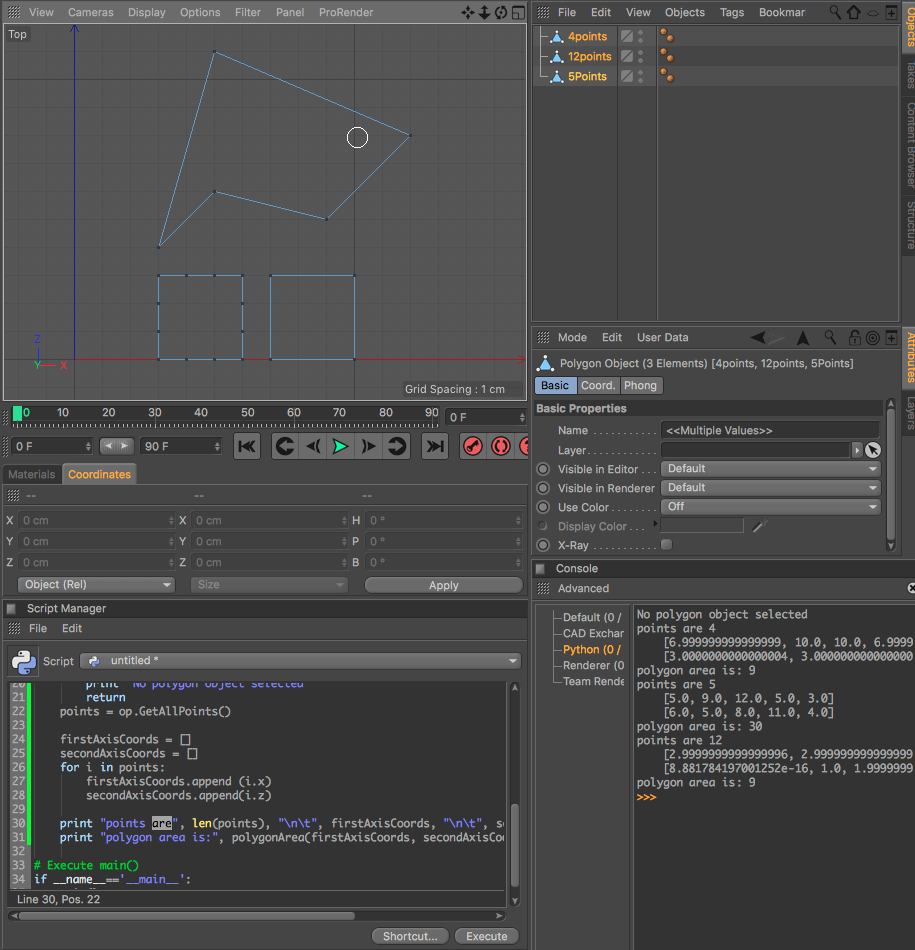# SOLVEDCompute the Area of an Irregular Polygon?

Hi,

I'm trying to model a floorplan. And to be dead-accurate, I'm thinking if there is a way to compute an area of an irregular polygon?

This is a sample but I guess something like this.
https://www.dropbox.com/s/rfa5p36n3dtiqcc/c4d081_area_irregular_shape.JPG?dl=0

Thank you for looking at my problem.

Hi @bentraje,

you can caluculate the area as normal polygons, if you use GetNGonTranslationMap(). So you have a list of regular polygons.
To calculate a polygon area, simply use some kind of this function:

``````# Calculate face area
def CalculateFaceArea(op, polyIndex):
poly = op.GetPolygon(polyIndex)
v0 = op.GetPoint(poly.a)
v1 = op.GetPoint(poly.b)
v2 = op.GetPoint(poly.c)
v3 = op.GetPoint(poly.d)

if poly.IsTriangle():
return (0.5 * ((v1-v0) % (v2-v0)).GetLength())
return (0.5 * ((v1-v0) % (v2-v0))).GetLength() + (0.5 * ((v3-v2) % (v0-v2)).GetLength())
``````

Hi Bentraje,

with regard to computing the area of a non self-intersecting planar polygon you can use:

``````# area of a polygon using
# shoelace formula

# (X[i], Y[i]) are coordinates of i'th point.

def polygonArea(X, Y, n):
# Initialze area
area = 0.0

# Calculate value of shoelace formula
j = n - 1
for i in range(0,n):
area += (X[j] + X[i]) * (Y[j] - Y[i])
j = i   # j is previous vertex to i
# Return absolute ;
return int(abs(area / 2.0))
``````

Best, Riccardo

@mp5gosu

Thanks for the response, but for some reason, it only works in quads. You can see my test here where it breaks:
https://www.dropbox.com/s/en84oigny07a1vb/c4d081_area_irregular_shape_v2.JPG?dl=0

@r_gigante

Thanks for the response. The shoelace formula was new to me and it was interesting after reading some explanation.
Unfortunately, for some reason it breaks.
It works with a 4 point plane, but not with a 16-point plane.

Here is the revised code I used

``````import c4d

x = []
y = []

def main():
baseVector = op.GetAllPoints()

for vector in baseVector:
x.append(vector)
y.append(vector)

def polygonArea(X, Y, n):
# Initialze area
area = 0.0

# Calculate value of shoelace formula
j = n - 1
for i in range(0,n):
area += (X[j] + X[i]) * (Y[j] - Y[i])
j = i   # j is previous vertex to i
# Return absolute ;
return int(abs(area / 2.0))

if __name__=='__main__':
main()
print polygonArea(x, y, 16)

``````

You can also check the 4 point and 16 point plane here:
https://www.dropbox.com/s/6qfhy9yw3l3xdil/c4d081_area_irregular_shape.c4d?dl=0

The area (since this is a square) should result to 160,000 cm2

Thank you for looking at the problem.

Hi Bentraje, thanks for following up and sorry for coming late here.

I've checked your geometry and the issue is not with the algorithm, but in both cases with the geometries you're using to compute the area who don't shown vertexes being properly "ordered".
The algorithm I've briefly presented requires the vertexes to be ordered as if you're visiting them by walking on the outer edge of your n-gon or polygon in CCW or CW. It is then mandatory to generate an ordered list of vertexes before passing the data to the function to get the correct result.In order to come out with such an ordered list I warmly recommend you to visit the following old-threads:

Best, Riccardo